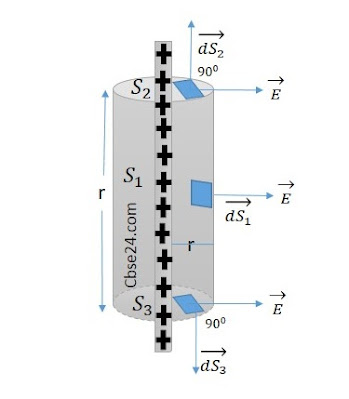# Electric field due to an infinitely long charge wire( Applying Gauss's theorem) | cbse24.com

### Electric field due to an infinitely long straight charged wire:

Consider a thin infinitely long straight wire having a uniform linear charge density λ C/m. The field Ē of the line charge is directed radially outwards and its magnitude is the same at all points equidistance from the line charge, we choose a cylindrical gaussian surface of radius r, length l and with the axis along with with the line charge. It has curved surface S1 and flat S2 and S3.

So only the curved surface contributes towards the total flux.Cylindrical Gaussian surface for a line charge

ϕE=.SE⃗ .dS=S1E⃗ .dS1+S2E⃗ .dS2+S3E⃗ .dS3

=S1E.dS1cos00+S2E.dS2cos900+S3E.dS3cos900

=E  ∫dS1+0+0

= E x area of the curved surface

ϕE=E×2πrl

Charge enclosed by the Gaussian surface, q=λl
Using Gauss's theorem ϕE=q/ε0

so, E.2πrl=λl/εo    or

E=λ2πϵ0r

Thus the electric field of a line charge is inversely proportional to the distance from the line charge.

*